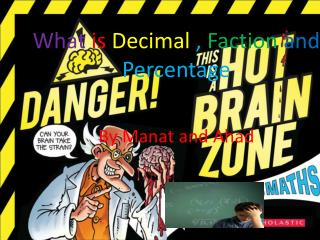DownloadDownload PresentationWhat is Decimal , Faction and Percentage

What is Decimal , Faction and Percentage

Télécharger la présentationWhat is Decimal , Faction and Percentage

- - - - - - - - - - - - - - - - - - - - - - - - - - - E N D - - - - - - - - - - - - - - - - - - - - - - - - - - -
Presentation Transcript

1. ./ What is Decimal , Faction and Percentage By Manat and Ahad

2. Content what is fraction Example Fraction Decimal Percentage Games for learning Games Quiz of the day Bye bye everyone

3. What is Decimal , Faction and Percentage Example Example one This is one of the example for Decimal Fraction and Percentage

4. What is Fraction? Fractions can be confusing sometimes but if you know how to do fractions it wouldn’t be that confusing. Everyone get mixed up doing Fractions even sometime teachers get mixed up. Here are some of the example for Fractions This is A Fraction of pizza If you slice a pizza you will get a Fraction .

5. What is Decimal A Decimal point goes between units and tenths and a units is ones and tenths is tens. And Decimals are sometimes confusing like I said before so check your answers twice. 45.6 has 4 tens, 5 units and 6 tenths, like this:

6. Percentages/Precent • There are many ways to explain about Percentages. If you pour 1 quarter water in a glass you will add 25% water to the glass. • If your Downloading something you would see how many Precent it has loaded.

7. http://www.sheppardsoftware.com/mathgames/fractions/FractionsToDecimals.htmhttp://www.sheppardsoftware.com/mathgames/fractions/FractionsToDecimals.htm http://www.mathplayground.com/Decention/Decention.html

8. of the Day http://www.math-play.com/Fractions-Decimals-Percents-Jeopardy/fractions-decimals-percents-jeopardy.html

9. Bye Bye Everyone Bye Bye Everyone